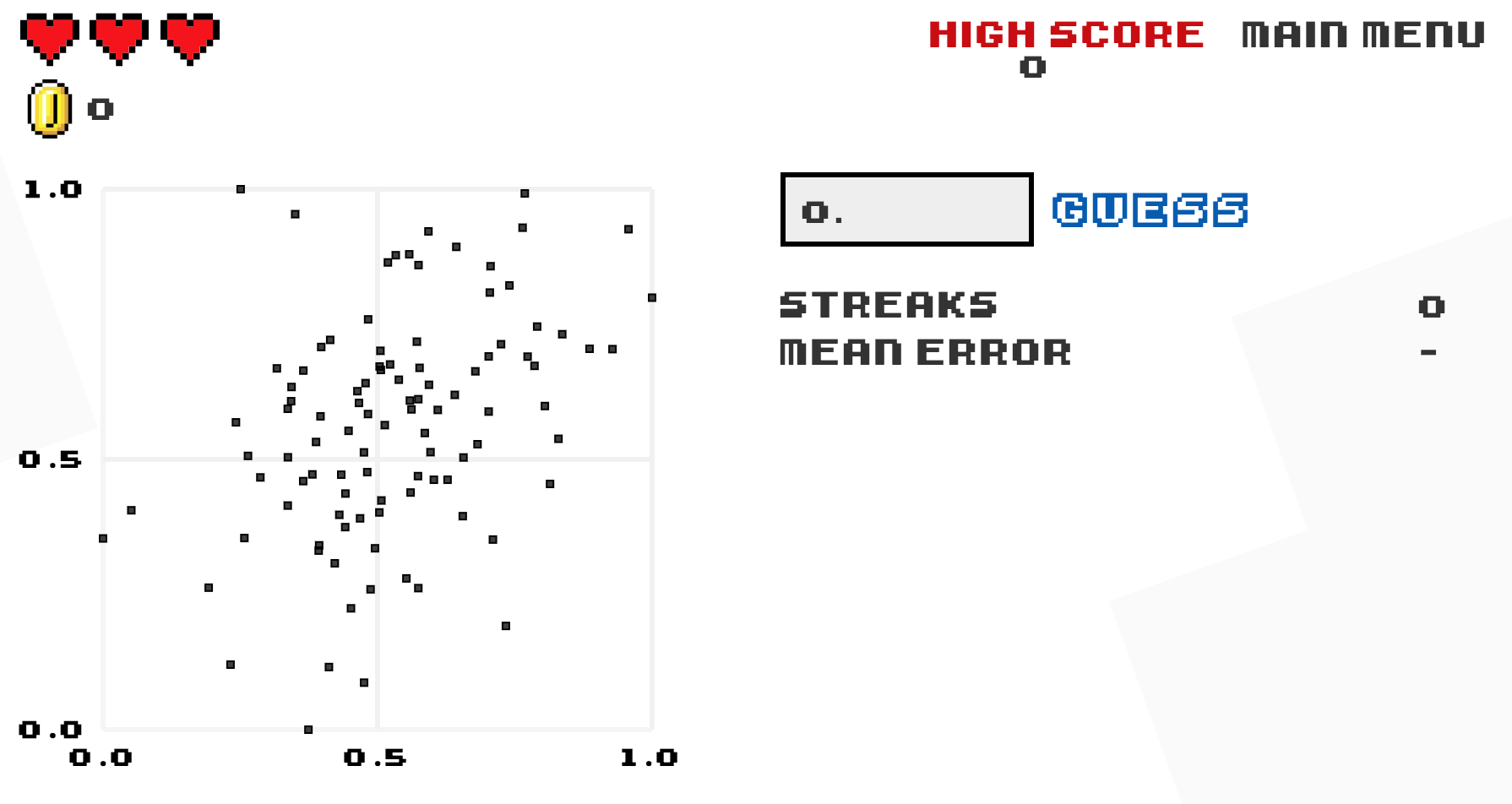## 5.4 ConclusionIn this chapter, you’ve studied the term basic regression, where you fit models that only have one explanatory variable. In Chapter 6, we’ll study multiple regression, where our regression models can now have more than one explanatory variable! In particular, we’ll consider two scenarios: regression models with one numerical and one categorical explanatory variable and regression models with two numerical explanatory variables. This will allow you to construct more sophisticated and more powerful models, all in the hopes of better explaining your outcome variable $$y$$.